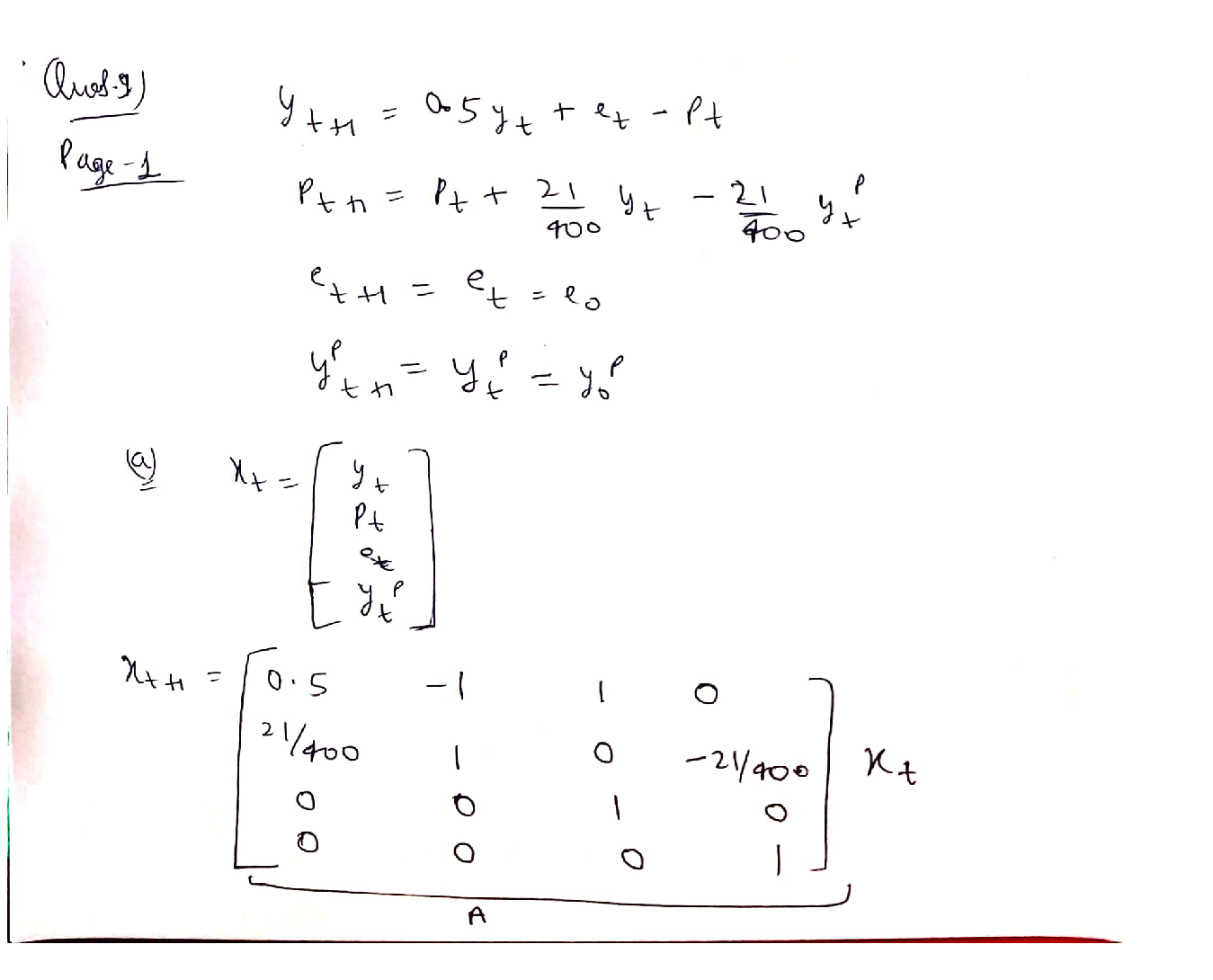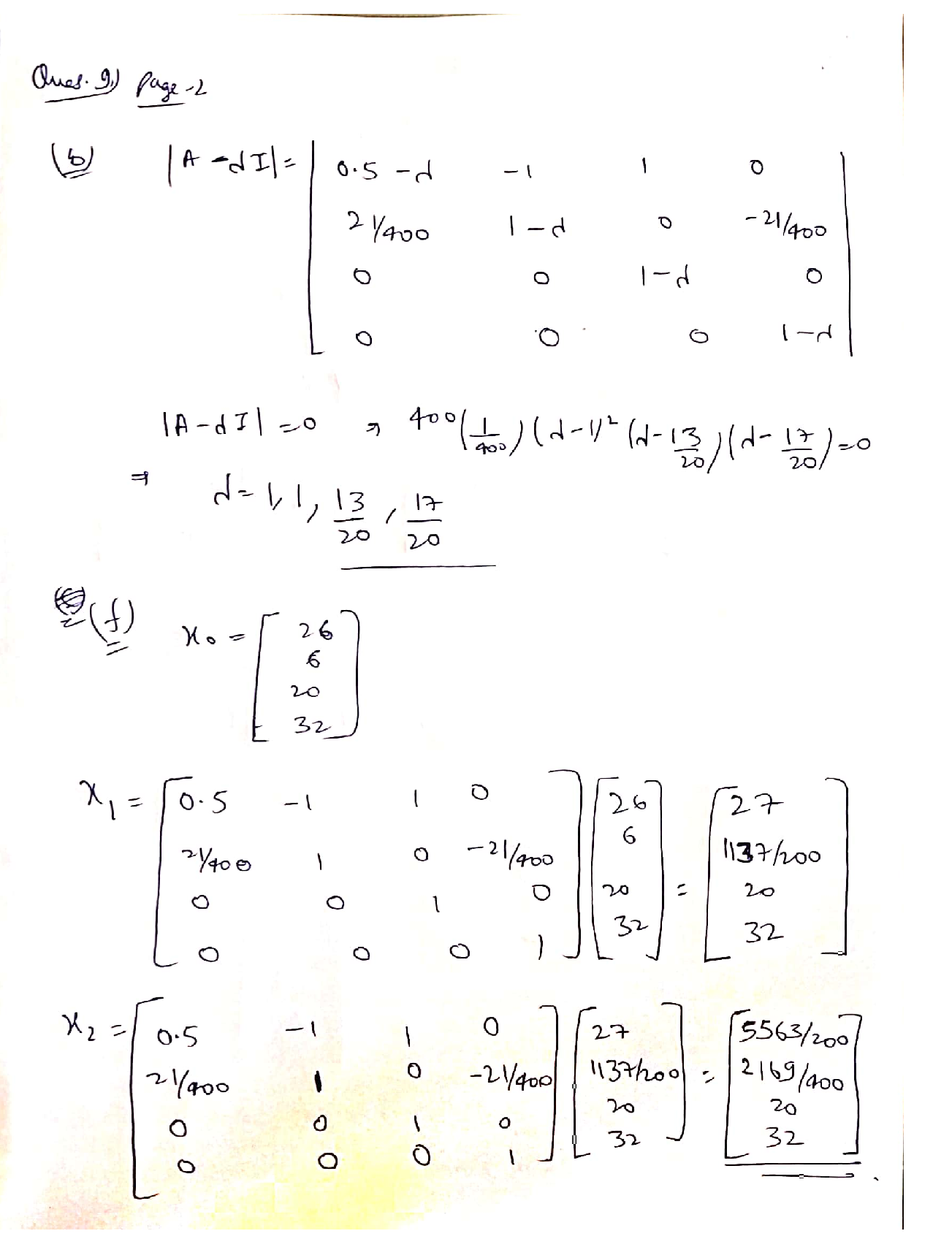Question

Linear Algebra

9. Consider the following linear, dynamic AS/AD model of real aggregate income (y) and the price of goods (p). Note that eo (autonomous expenditure) and yo^p(potential GDP) are both exogenously given and assumed to be strictly positive.

\begin{aligned} &\begin{aligned} -y_{t+1} &=0.5 y_{t}+e_{t}-p_{t} \\ -& p_{t+1}=p_{t}+(21 / 400)\left(y_{t}-y_{t}^{p}\right) \end{aligned}\\ &\boldsymbol{e}_{\boldsymbol{t}+\mathbf{1}}=\boldsymbol{e}_{\boldsymbol{t}}=\boldsymbol{e}_{\boldsymbol{0}}\\ &y_{t+1}^{p}=y_{t}^{p}=y_{0}^{p} \end{aligned}

\text { a. Letting } x_{t}=\left[\begin{array}{llll} y_{t} & p_{t} & e_{t} & y_{t}^{p} \end{array}\right]^{T}, \text { write down the } 4 \text { by } 4 \text { matrix } A, \text { for which } A x_{t}=x_{t+1}

b. Find all of A's eigenvalues.

c. What do the eigenvalues of A tell you about the steady-state equilibrium for this model?

d. Find the steady-state equilibrium vector, x*, expressed as a function of its exogenous determinants (eo and y).

e. All else equal, how would an increase in autonomous expenditure affect the steady-state values of y and p? Explain.

f. Suppose that at time 0, xo= [26 6 20 32]T. What would y and p be at time 1? At time 2?Verified### Question 52404Linear Algebra

In an election campaign, the popularity, B, as a percent of voters, of the governing Blue party can be modelled by a function of time, t, in days throughout the campaign as B(t) = 40 - 0.5t. The popularity, R(t), of the opposing Red party can be modelled by a composite function of B(t),R(B(t)) = 20 + 0.75[40 – B(t)].
Graph B(t) and describe the trend.

### Question 45665Linear Algebra

\text { 4] Find } g(0)-g(9)+g(2) \text {, if }
g(x)=\left\{\begin{aligned} \frac{x+1}{2}, & \text { if } x \text { is odd } \\ \frac{x}{2}, & \text { if } x \text { is even } \end{aligned}\right.

### Question 45664Linear Algebra

\text { 3] Find } f(4)-f(2)+f(3) \text {, if }
f(x)=\left\{\begin{array}{ll} \frac{x+1}{2}, & \text { if } x \text { is odd } \\ \frac{x}{4}, & \text { if } x \text { is even } \end{array}\right.

### Question 45663Linear Algebra

2] Find the Domains of the following functions:
\text { a) } f(x)=\sqrt{15-5 x}
f(x)=\frac{x^{2}-2 x+1}{x^{2}-4 x-21}
f(x)=\frac{x^{2}-2 x+1}{\sqrt{16-2 x}}

### Question 45662Linear Algebra

a) Give the definition of a rational function. [5 pts]
b) Give an example of a polynomial function of degree 3. [5 pts]
c) Can a constant function be a polynomial and a rational function at the same time? Explain your answer. [5 pts]
d) Give an example of a non-polynomial function and explain why not apolynomial function. [10 pts)

### Question 45333Linear Algebra

5) Ве,
\begin{array}{c} f: \mathbb{R} \rightarrow \mathbb{R} \\ f(x)=\left\{\begin{array}{ll} x^{2}-3 \cos (\pi x) & x<0 \\ x-4 e^{-2 x} & x \geq 0 . \end{array}\right. \end{array}
Calculate
\int_{-1}^{2} f(x) d x
Presenting the result in simplified form

### Question 45332Linear Algebra

4) Be f: R - Ra function differentiable in R such your derivative f', has in maximum a real zero.
Prove that the equation f(x)=0 has in maximum 2 real square

### Question 45331Linear Algebra

3) Take in consideration the following function
f(x)=\left\{\begin{array}{ll} f: \mathbb{R} \rightarrow \mathbb{R} & \\ \frac{x^{2}-4 x+\cos (\sin (x)),}{x^{4}+4 x^{2}+1}, & x \leq 0 \end{array}\right.
a) Show that the function f is continuous in R+ and in R- but discontinuous in the point X
b) Say justifying, if f is differenciable in X=0

### Question 45330Linear Algebra

2) Prove by definition that
\lim _{x \rightarrow 0} x^{2} \cos \left(e^{x}\right)=0

### Question 45329Linear Algebra

1) Calculate the following limit:
\lim _{x \rightarrow+\infty} \frac{x^{2}\left(e^{-3 x}+1\right)+x \cos (5 x)}{x^{2}+7 x+1}

### Submit query